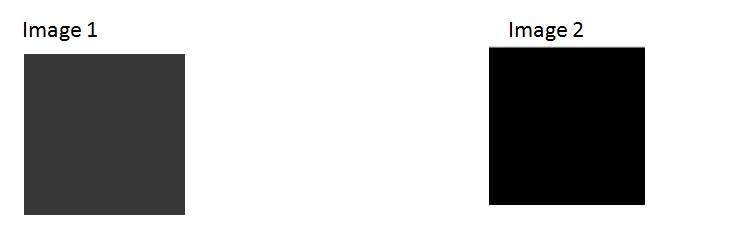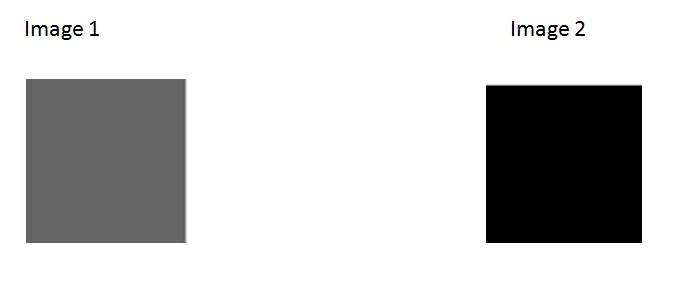# Brightness and Contrast

## Brightness:

Brightness is a relative term. It depends on your visual perception. Since brightness is a relative term , so brightness can be defined as the amount of energy output by a source of light relative to the source we are comparing it to. In some cases we can easily say that the image is bright , and in some cases, its not easy to perceive.

### For example:

Just have a look at both of these images , and compare which one is brighter.We can easily see , that the image on the right side is brighter as compared to the image on the left.

But if the image on the right is made more darker then the first one , then we can say that the image on the left is more brighter then the left.

## How to make an image brighter.

Brightness can be simply increased or decreased by simple addition or subtraction, to the image matrix.

Consider this black image of 5 rows and 5 columnsSince we already know, that each image has a matrix at its behind that contains the pixel values. This image matrix is given below.

 0 0 0 0 0 0 0 0 0 0 0 0 0 0 0 0 0 0 0 0 0 0 0 0 0

Since the whole matrix is filled with zero, and the image is very much darker.

Now we will compare it with another same black image to see this image got brighter or not.Still both the images are same, now we will perform some operations on image1 , due to which it becomes brighter then the second one.

What we will do is , that we will simply add a value of 1 to each of the matrix value of image 1. After adding the image 1 would something like this.Now we will again compare it with image 2 , and see any difference.We see, that still we cannot tell which image is brighter as both images looks the same.

Now what we will do , is that we will add 50 to each of the matrix value of the image 1 and see what the image has become.

The output is given below.Now again , we will compare it with image 2.Now you can see that the image 1 is slightly brighter then the image 2. We go on , and add another 45 value to its matrix of image 1 , and this time we compare again both images.Now when you compare it , you can see that this image1 is clearly brighter then the image 2.

Even it is brighter then the old image1. At this point the matrix of the image1 contains 100 at each index as first add 5 , then 50 , then 45. So 5 + 50 + 45 = 100.

## Contrast

Contrast can be simply explained as the difference between maximum and minimum pixel intensity in an image.

### For example.

Consider the final image1 in brightness.The matrix of this image is:

 100 100 100 100 100 100 100 100 100 100 100 100 100 100 100 100 100 100 100 100 100 100 100 100 100

The maximum value in this matrix is 100.

The minimum value in this matrix is 100.

Contrast = maximum pixel intensity(subtracted by) minimum pixel intensity

= 100 (subtracted by) 100

= 0

0 means that this image has 0 contrast.